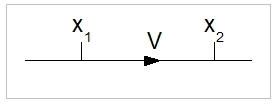# Distance, Speed & Acceleration

## Speed

Speed is defined as:

v = dx /dt

where:
v : speed in m/s
x : distance in metres (m)
t : time in seconds (s)### Explanation

The speed of on object equals the distance travelled per unit of time.

### Examples

A helicopter is travelling at a speed of 50 m/s. How long will it take to travel a distance of 100 nautical miles, assuming an constant speed?
Answer: One nautical mile equals 1,852 m. Thus, 100 nautical miles is 185,200 metres. The time needed follows from t = x / v, thus 185,200 / 50 =  3,704 seconds, or 61.7 minutes.

## Acceleration

Acceleration is defined as:

a = dv / dt

Where:
a : acceleration in m/s2
v : speed in m/s
t : time in seconds (s)

### Explanation

The acceleration of an object equals the change of speed per unit of time.

### Examples

A helicopter starts from a hover and commences a horizontal flight. In 10 seconds, it attains a horizontal speed of 20 m/s. What is the average acceleration?
Answer: Horizontal speed in the hover is 0 m/s, thus the change of speed is 20 m/s. Acceleration is 20 (m/s) /10 (s) = 2 m/s2.

The helicopter now starts decelerating at a rate of 3 m/s2. How long will it take it to get to a speed of zero?
Answer: dt = dv/a = 20 / 3 = 6.67 seconds.

## Distance / Position

When we know the speed and acceleration of an object, we can calculate the position at time t. We will now consider the equation involved.

Position at time t:

x(t) = I(v) d t

( I(t) is the notation we use for the integral over time t)

Assuming a constant acceleration:

v = v0 + at

Combining: x(t) = I(v0 + at)dt= I(t) - I(0) = V0.t + (1/2) . at2 + C

C has the meaning of the distance at time 0. So we denote C as x0.

To summarise: x(t) = x0 + v0.t +(1/2).at2

### Explanation

The position of an object at time t is defined by its default position, velocity, and acceleration at time t = 0 (with a constant acceleration, thus the force working on the object is constant).

### Example1

A helicopter flies at a horizontal speed of 0 m/s while accelerating at a rate of 2 m/s2. We define this as the starting situation (time t=0). How long will it take it to travel 1.6 km (about one mile)? And what is its speed after it has travelled this distance?
x(t) = x0 + v0.t +(1/2).at2
x(t) = 1600 (m)
v0= 0 (m/s)
a = 2 m/s2
x0=0

Substitute these values to get:

1600 = 0 + 0 . t +(1/2) . 2t2
t2  = 1600
t = 40 s.

The speed is then : v=a.t = 2.40 = 80 m/s. Note that this is really rather fast (80 m/s = 288 km/s = 180 miles/hour)!

### Example2

Another example: A stone is falling to earth from a height of 1524 m (5000 feet). How long will it take it to reach the earth's surface (surface at 0 feet and no friction assumed)? Gravity acceleration is 9.8 m/s2.
1524 = (1/2)  9.8  t2= 4.9 t2
t2 = 1524 / 4.9
t = 17.6 s

# Do you want to comment this topic?

1: (Book) Cyclic and Collective2: (Book) Principles of Helicopter Flight4: Logitech Extreme 3D Pro Joystick5: Saitek Pro Flight Rudder Pedals